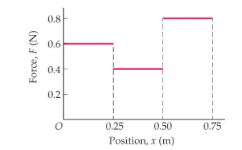# Problem: The force shown in the figure (Figure 1) moves an object from x = 0 to x = 0.75 m. How much work is done by the force?

###### FREE Expert Solution

In position-force graphs, work done is calculated from the area under the curve.

We'll sum up the area under the three shapes from x = 0.00 m to 0.75m.###### Problem Details

The force shown in the figure (Figure 1) moves an object from x = 0 to x = 0.75 m.
How much work is done by the force?